## Detect Changes in Data and Expression Values

Stateflow® charts can detect changes in the values of data and expressions between time steps. You can:

• Use change detection operators to determine when a variable changes to or from a value.

• Use edge detection operators to determine when an expression rises above or falls below a threshold.

To generate an implicit local event when the chart sets the value of a variable, use the `change` operator. For more information, see Control Chart Behavior by Using Implicit Events.

### Change Detection Operators

To detect changes in Stateflow data, use the operators listed in this table.

OperatorSyntaxDescriptionExample
`hasChanged``tf = hasChanged(data_name)`Returns 1 (`true`) if the value of `data_name` at the beginning of the current time step is different from the value of `data_name` at the beginning of the previous time step. Otherwise, the operator returns 0 (`false`).

Transition out of state if any element of the matrix `M` has changed value since the last time step or input event.

`[hasChanged(M)]`

Transition out of state if the element in row 1 and column 3 of the matrix `M` has changed value since the last time step or input event.

In charts that use MATLAB® as the action language, use:

`[hasChanged(M(1,3))]`

In charts that use C as the action language, use:

`[hasChanged(M)]`
`hasChangedFrom``tf = hasChangedFrom(data_name,value)`Returns 1 (`true`) if the value of `data_name` was equal to the specified `value` at the beginning of the previous time step and is a different value at the beginning of the current time step. Otherwise, the operator returns 0 (`false`).

Transition out of state if the previous value of the structure `struct` was equal to `structValue` and any field of `struct` has changed value since the last time step or input event.

`[hasChangedFrom(struct,structValue)]`
`hasChangedTo``tf = hasChangedTo(data_name,value)`Returns 1 (`true`) if the value of `data_name` was not equal to the specified `value` at the beginning of the previous time step and is equal to `value` at the beginning of the current time step. Otherwise, the operator returns 0 (`false`).

Transition out of state if the structure field `struct.field` has changed to the value `5` since the last time step or input event.

`[hasChangedTo(struct.field,5)]`

Note

If multiple input events occur in the same time step, these operators can detect changes in data value between input events.

#### Example of Chart with Change Detection

This model shows how the operators `hasChanged`, `hasChangedFrom`, and `hasChangedTo` detect specific changes in an input signal. In this example, a Ramp (Simulink) block sends a discrete, increasing time signal to a chart.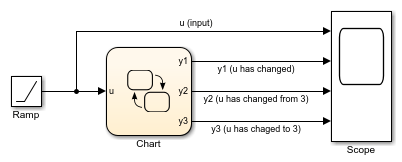The model uses a fixed-step solver with a step size of 1. The signal increments by 1 every time step. The chart analyzes the input signal `u` for these changes:

• Any change from the previous time step

• A change to the value 3

• A change from the value 3

To check the signal, the chart calls three change detection operators in a transition action. The chart outputs the return values as `y1`, `y2`, and `y3`.

During simulation, the Scope (Simulink) block shows the input and output signals for the chart.

• The value of `u` increases by 1 every time step.

• The value of `y1` changes from 0 to 1 at time `t = 1`. The value of `y1` remains 1 because `u` continues to change at each subsequent time step.

• The value of `y2` changes from 0 to 1 at time `t = 4` when the value of `u` changes from 3 to 4. The value of `y2` returns to 0 after one time step.

• The value of `y3` changes from 0 to 1 at time `t = 3` when the value of `u` changes from 2 to 3. The value of `y3` returns to 0 after one time step.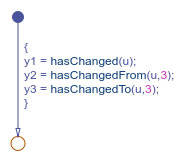#### Limitations of Change Detection

The type of Stateflow chart determines the scope of the data supported for change detection:

• Standalone Stateflow charts in MATLAB: `Local` only

• Charts in Simulink® that use MATLAB as the action language: `Input` only

• Charts in Simulink that use C as the action language: `Input`, `Output`, `Local`, or `Data Store Memory`

The argument `data_name` can be:

• A scalar variable.

• A matrix or an element of a matrix.

• If `data_name` is a matrix, the operator returns `true` when it detects a change in any element of `data_name`.

• Index elements of a matrix by using numbers or expressions that evaluate to a constant integer. See Operations for Vectors and Matrices in Stateflow.

• A structure or a field in a structure.

• If `data_name` is a structure, the change detection operator returns `true` when it detects a change in any field of `data_name`.

• Index fields in a structure by using dot notation. See Index and Assign Values to Stateflow Structures.

• Any valid combination of structure fields or matrix elements.

The argument `data_name` cannot be a nontrivial expression or a custom code variable.

Note

Standalone charts in MATLAB do not support change detection on an element of a matrix or a field in a structure.

For the `hasChangedFrom` and `hasChangedTo` operators, the argument `value` can be any expression that resolves to a value that is comparable with `data_name`.

• If `data_name` is a scalar, then `value` must resolve to a scalar value.

• If `data_name` is a matrix, then `value` must resolve to a matrix value with the same dimensions as `data_name`.

Alternatively, in a chart that uses C as the action language, `value` can resolve to a scalar value. The chart uses scalar expansion to compare `data_name` to a matrix whose elements are all equal to the value specified by `value`. See Assign Values to All Elements of a Matrix.

• If `data_name` is a structure, then `value` must resolve to a structure value whose field specification matches `data_name` exactly.

If you generate code from a chart that uses change detection operators and row-major array layout is enabled, code generation produces an error. Before generating code, enable column-major array layout. See Select Array Layout for Matrices in Generated Code.

### Edge Detection Operators

To determine when an expression rises above or falls below a threshold, use the operators listed in this table.

OperatorSyntaxDescriptionExample
`crossing``tf = crossing(expression)`

Returns 1 (`true`) if:

• The previous value of `expression` was positive and its current value is zero or negative.

• The previous value of `expression` was zero and its current value is nonzero.

• The previous value of `expression` was negative and its current value is zero or positive.

Otherwise, the operator returns 0 (`false`).

This operator imitates the behavior of a Trigger (Simulink) block with Trigger Type set to `either`.

Transition out of state if the value of the input data `signal` crosses a threshold of 2.5.

`[crossing(signal-2.5)]`

The edge is detected when the value of the expression `signal-2.5` changes from positive to negative, from negative to positive, from zero to nonzero, or from nonzero to zero.

`falling``tf = falling(expression)`

Returns 1 (`true`) if:

• The previous value of `expression` was positive and its current value is zero or negative.

• The previous value of `expression` was zero and its current value is negative.

Otherwise, the operator returns 0 (`false`).

This operator imitates the behavior of a Trigger (Simulink) block with Trigger Type set to `falling`.

Transition out of state if the value of the input data `signal` falls below a threshold of 2.5.

`[falling(signal-2.5)]`

The falling edge is detected when the value of the expression `signal-2.5` changes from positive to negative, from positive to zero, or from zero to negative.

`rising``tf = rising(expression)`

Returns 1 (`true`) if:

• The previous value of `expression` was negative and its current value is zero or positive.

• The previous value of `expression` was zero and its current value is positive.

Otherwise, the operator returns 0 (`false`).

This operator imitates the behavior of a Trigger (Simulink) block with Trigger Type set to `rising`.

Transition out of state if the value of the input data `signal` rises above a threshold of 2.5.

`[rising(signal-2.5)]`

The rising edge is detected when the value of the expression `signal-2.5` changes from negative to positive, from negative to zero, or from zero to positive.

Note

Like the Trigger block, these operators detect a single edge when the `expression` argument changes value from positive to zero to negative or from negative to zero to positive at three consecutive time steps. The edge occurs when the value of the expression becomes zero.

#### Example of Chart with Edge Detection

This model shows how the operators `crossing`, `falling`, and `rising` detect edges in an input signal. In this example, a Pulse Generator (Simulink) block sends a square wave to a chart.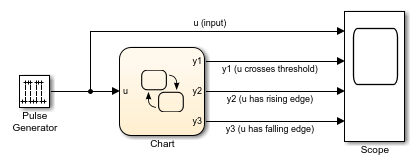The model uses a fixed-step solver with a step size of 1. The value of the input signal `u` alternates between 0 and 5 every two time steps. The chart analyzes the input signal `u` for these edges:

• A falling or rising edge crossing the threshold of 2.5

• An edge rising over the threshold of 2.5

• An edge falling under the threshold of 2.5

To check the signal, the chart calls three edge detection operators in a transition action. The chart outputs the return values as `y1`, `y2`, and `y3`.

During simulation, the Scope (Simulink) block shows the input and output signals for the chart.

• The value of `u` alternates between 0 and 5 at every other time step.

• The value of `y1` changes from 0 to 1 at time `t = 1`, `3`, `5`, and `7`, when the value of the expression `u-2.5` changes sign. The value of `y1` returns to 0 after one time step.

• The value of `y2` changes from 0 to 1 at time `t = 1` and `5`, when the value of the expression `u-2.5` changes from negative to positive. The value of `y2` returns to 0 after one time step.

• The value of `y3` changes from 0 to 1 at time `t = 3` and `7`, when the value of the expression `u-2.5` changes from positive to negative. The value of `y3` returns to 0 after one time step.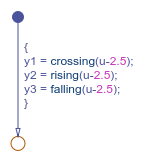#### Limitations of Edge Detection

Edge detection is supported only in Stateflow charts in Simulink models.

The argument `expression`:

• Must be a scalar-valued expression

• Can combine chart input data, constants, nontunable parameters, continuous-time local data, and state data from Simulink based states

• Can include addition, subtraction, and multiplication of scalar variables, elements of a matrix, fields in a structure, or any valid combination of structure fields and matrix elements

Index elements of a matrix by using numbers or expressions that evaluate to a constant integer.

Edge detection for continuous-time local data and state data from Simulink based states is supported only in transition conditions.

In atomic subcharts, map all input data that you use in edge detection expressions to input data or nontunable parameters in the main chart. Mapping these input data to output data, local data, or tunable parameters can result in undefined behavior.

Stateflow charts that use edge detection operators do not support operating points.

### Implementation of Change and Edge Detection

A chart detects changes in chart data and expressions by evaluating the values at time step boundaries. The chart compares the value at the beginning of the previous execution step with the value at the beginning of the current execution step.

For example, when you invoke the `hasChanged` operator with an argument of `x`, the Stateflow chart double-buffers the values of `x` in local variables.

Local BufferDescription
`x_prev`

Value of data `x` at the beginning of the last time step

`x_start`

Value of data `x` at the beginning of the current time step

To detect changes, the chart double-buffers data values after an event triggers the chart but before the chart begins execution. If the values of `xprev` and `xstart` match, the change detection operator returns `false` to indicate that no change occurred; otherwise, it returns `true` to indicate a change. This diagram places these tasks in the context of the chart life cycle.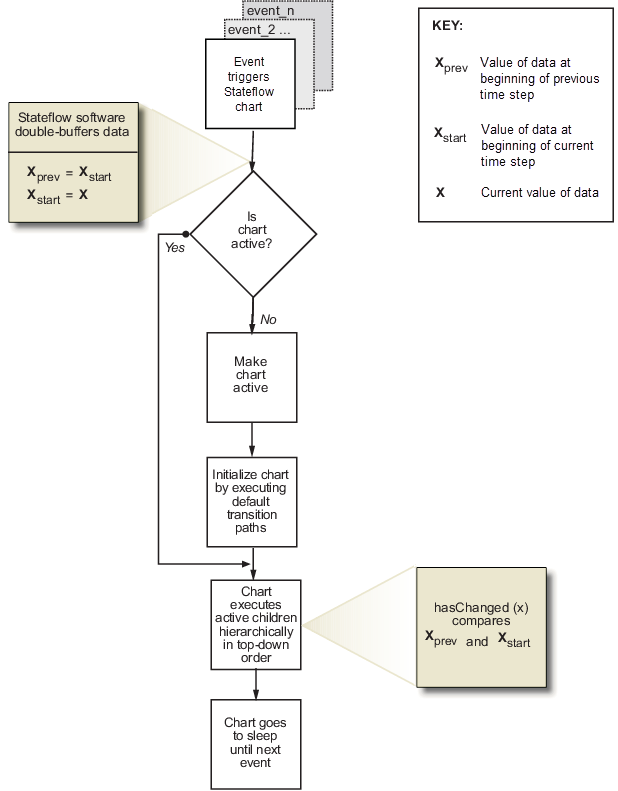Edge detection operators behave in a similar way, except that they compare the value of an expression at the beginning of the last time step (`xprev`) with its current value (`x`). The difference in implementation allows continuous-time charts to detect edges in local data during minor time steps.

#### Transient Value Changes in Local Data

The change detection operators attempt to filter out transient changes in local chart variables by evaluating their values only at time boundaries. The chart evaluates the specified local variable only once at the end of the execution step. The return value of the change detection operators remains constant even if the value of the local variable fluctuates within a given time step. For example, suppose that in the current time step, the local variable `temp` changes from its value at the previous time step but then reverts to the original value. The operator `hasChanged(temp)` returns `false` for the next time step, indicating that no change occurred.

In contrast, the edge detection operators can detect edges in continuous-time local data during minor time steps. For example, suppose that `p` is a continuous-time local variable with a negative derivative. Then the operator `falling(p)` returns `true` during the minor time step when `p` changes sign from positive to negative.

#### Detect Value Changes Between Input Events or Super Step Iterations

When multiple input events occur in the same time step, or when you enable super step semantics, the chart updates the `xprev` and `xstart` buffers every time it executes. The chart detects changes in value between input events and super step iterations even if the changes occur more than once in a given time step. For more information, see Use Events to Execute Charts and Super Step Semantics.